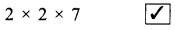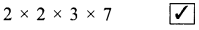# DAV Class 5 Maths Chapter 3 Worksheet 8 Solutions

The DAV Class 5 Maths Book Solutions Pdf and DAV Class 5 Maths Chapter 3 Worksheet 8 Solutions of Multiples and Factors offer comprehensive answers to textbook questions.

## DAV Class 5 Maths Ch 3 Worksheet 8 Solutions

Question 1.
(a) Prime factorisation of 28 isSolution:(b) Prime factorisation of 42 isSolution:(c) Prime factorisation of 36 isSolution:(d) Prime factorisation of 84 isSolution:Question 2.
State the answer in Yes or No.
(a) The prime factorisation of 15 is 3 × 5. _______
Solution:
Yes

(b) The prime factorisation of 50 is 2 × 5 × 5. _______
Solution:
Yes

(c) The prime factorisation of 90 is 2 × 5 × 9. _________
Solution:
No
Prime factorisation of 90 : 2 × 3 × 3 × 5(d) The prime factorisation of 99 is 3 × 3 × 11. _______
Solution:
Yes

(e) The prime factorisation of 63 is 7 × 9. ________
Solution:
No
Prime factorisation of 63 : 3 × 3 × 7

(f) The prime factorisation of 54 is 2 × 27. ________
Solution:
No
Prime factorisation of 54 : 2 × 3 × 3 × 3

DAV Class 5 Maths Chapter 3 Worksheet 8 Notes

15 can be written in different ways.
15 = 1 × 15
15 = 1 × 3 × 5
All the factors are not prime.
15 = 3 × 5 → Factors are prime only.
Factorisation in which every factor is prime is called the Prime factorisation of the number.